Latest Banking jobs   »   Reasoning Ability Quiz For IBPS RRB...

# Reasoning Ability Quiz For IBPS RRB PO, Clerk Prelims 2021- 11th June

Directions (1-5): Read the following information carefully and answer the questions given below.
In a certain code language:
“Inside result happy spirit” is written as “ae me le ue”.
“Picture down morning inside” is written as “de le te ge”.
“Happy picture good spirit” is written as “te ae ue ce”.
“Spirit win morning spark” is written as “de ye we ae”.

Q1. What is the possible code for “good way down”?
(a) ce me ge
(b) pe me ge
(c) ce te le
(d) pe ce ge
(e) None of these

Q2. If “Inside win picture” is coded as “ye le te”, then what will be the code for “Spark”?
(a) xe
(b) we
(c) ce
(d) de
(e) Can’t determined

Q3. What is the code for “Morning”?
(a) de
(b) te
(c) ae
(d) ue
(e) None of these

Q4. What is the word for the code “me” in the given code language?
(a) spirit
(b) good
(c) result
(d) spark
(e) win

Q5. If “Spirit give happy” is written as “ue ae re”, then what will be the code for “Give”?
(a) ae
(b) ue
(c) re
(e) Can’t determine

Directions (6-10): Study the following information carefully and answer the given questions:
In a certain code language,
“only in serial order” is written as “ve pu na to”,
“order in the state” is written as “li ve su pu”,
“the logical idea only” is written as “su na ri jo”
“in idea or theory” is written as “zt jo bk pu”

Q6. Which of the following is the code of ‘or’?
(a) zt
(b) bk
(c) jo
(d) pu
(e) either ‘zt’ or ‘bk’

Q7. The code ‘li ri to ve’ may represent:
(a) serial order theory only
(b) only idea state order
(c) state logical serial order
(d) serial theory state the
(e) only the idea logical

Q8. Which of the following may represent ‘logical idea is order’?
(a) jo na ri ge
(b) ve na ri jo
(c) ri ve na zt
(d) bk to pu jo
(e) None of these

Q9. Which of the following is the code of ‘only’?
(a) su
(b) jo
(c) na
(d) ri
(e) Cannot be determined

Q10. Which of the following is code of ‘theory’?
(a) zt
(b) bk
(c) ve
(d) su
(e) Cannot be determined

Directions (11-15): Study the following information carefully and answer the questions given below:
In a certain code language
‘study book room rent’ is coded as ‘dy bk rm rt’
‘room rent is high’ is coded as ‘rm rt si gh’
‘pay rent is more ’ is coded as ‘yp rt si me’
‘more study work hard’ is coded as ‘me dy wk hd’

Q11. What is the code for ‘more room’?
(a) rm si
(b) me gh
(c) me rm
(d) bk rm
(e) None of these

Q12. What is the code for ‘work is hard’?
(a) hd gh si
(b) wk hd rt
(c) wk si hd
(d) si wk yp
(e) Cannot be determined

Q13. ‘dy’ is denoted as?
(a) book
(b) room
(c) rent
(d) study
(e) None of these

Q14. What is the code for ‘book’?
(a) dy
(b) bk
(c) rt
(d) rm
(e) None of these

Q15. ‘wk’ is the code for?
(a) work
(b) high
(c) hard
(d) Either (a) or (b)
(e) Cannot be determined

Practice More Questions of Reasoning for Competitive Exams:

###### Study Plan for IBPS RRB PO/Clerk Prelims 2021

Solutions

Solution (1-5):
Sol.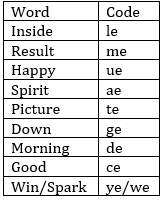S1. Ans(d)
S2. Ans(b)
S3. Ans(a)
S4. Ans(c)
S5. Ans(c)

Solutions (6-10):
Sol.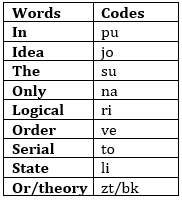S6. Ans.(e)
S7. Ans.(c)
S8. Ans.(e)
S9. Ans.(c)
S10. Ans.(e)

Solutions (11-15):
Sol.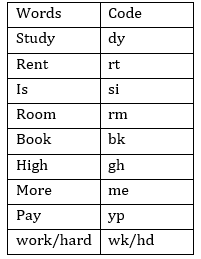S11. Ans.(c)
S12.Ans.(c)
S13.Ans.(d)
S14.Ans.(b)
S15.Ans.(e)#### Congratulations!Incorrect details? Fill the form again here

•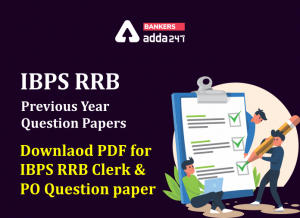IBPS RRB Previous Year Question Paper PD...
•Reasoning Ability Quiz For IBPS RRB PO C...
•Reasoning Ability Quiz For IBPS Clerk Pr...
•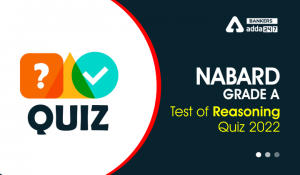Reasoning Ability Quiz For NABARD Grade ...
•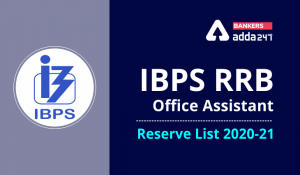IBPS RRB Clerk 2nd Reserve List 2022 Out...
•Reasoning Ability Quiz For IBPS Clerk Pr...